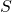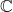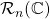# Characterization of ideals of rhotrices over a ring and its applications

Kailash M. Patil
Notes on Number Theory and Discrete Mathematics
Print ISSN 1310–5132, Online ISSN 2367–8275
Volume 27, 2021, Number 1, Pages 138—147
DOI: 10.7546/nntdm.2021.27.1.138-147

## Details

### Authors and affiliations

Kailash M. PatilDepartment of Mathematics, Dharmsinh Desai University

### Abstract

We define higher order rhotrices over a commutative unital ringand obtain a ringof rhotrices of the order. We characterize the ideals and maximal ideals of. As a particular case, we record ideals of rhotrix rings over integers and rhotrix algebras over complex plane. As an application, we characterize the maximal ideals of the commutative unital Banach algebra.

### Keywords

• Rhotrix over a ring
• Unital ring
• Maximal ideal
• Banach algebra

• 15B99
• 46J20

### References

1. Ajibade, A. O. (2003). The concept of rhotrix in mathematical enrichment. International Journal of Mathematical Education in Science and Technology, 34(2), 175–179.
2. Atanassov, K. T., & Shannon, A. G. (1998). Matrix-Tertions and Matrix-Noitrets: Exercises in Mathematical Enrichment. International Journal of Mathematical Education in Science and Technology, 29, 898–903.
3. Bhatt, S. J., & Karia, D. J. (1998). A note on Banach algebras without order. Prajna Journal of Sardar Patel University, 8(1), 30–32.
4. Isere, A. O. (2018). Even dimensional rhotrix. Notes on Number Theory and Discrete Mathematics, 24(2), 125–133.
5. Isere, A. O. (2019). Representation of higher even-dimensional rhotrix. Notes on Number Theory and Discrete Mathematics, 25(1), 206–219.
6. Mohammed, A. (2009). A remark on the classifications of rhotrices as abstract structures. International Journal of Physical Sciences, 4(9), 496–499.
7. Mohammed, A. (2011). Theoretical Development and Applications of Rhotrices. PhD thesis, Ahmadu Bello University, Zaria.
8. Mohammed, A., & Okon, U. E. (2016). On subgroups of non-commutative general rhotrix group. Notes on Number Theory and Discrete Mathematics, 22(2), 72–90.
9. Patil, K. M., Singh, H. P., & Sutaria, K. A. (2015). The eigen values of any given 3×3 matrix via eigen values of its corresponding rhotrix. International Journal of Computer and Mathematical Sciences, 4(11), 1–4.
10. Sani, B. (2007). The row-column multiplication of high dimensional rhotrices. International Journal of Mathematical Education in Science and Technology, 38(5), 657–662.
11. Sani, B. (2008). Conversion of a rhotrix to a ‘coupled matrix’. International Journal of Mathematical Education in Science and Technology, 39(2), 244–249.
12. Sharma, P. L. & Kanwar, R. K. (2012). The Cayley–Hamilton theorem for rhotrices. International Journal Mathematics and Analysis, 4(1), 171–178.
13. Usaini, S. & Aminu, A. (2017). Exponential function of rhotrices. International Journal of Mathematics and Statistics, 18(1), 21–29.

## Cite this paper

Patil, K. M. (2021). Characterization of ideals of rhotrices over a ring and its applications. Notes on Number Theory and Discrete Mathematics, 27(1), 138-147, doi: 10.7546/nntdm.2021.27.1.138-147.